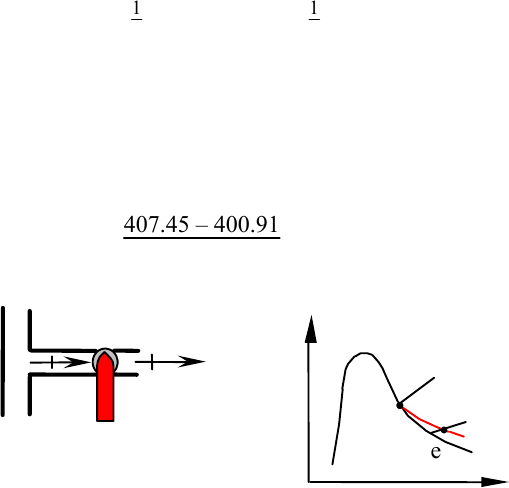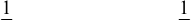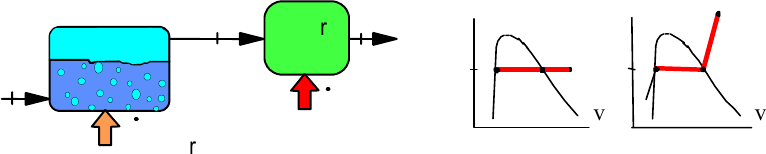Textbook Notes (290,000)
US (110,000)
LSU (20,000)
ME (300)
ME 3333 (50)
All (50)
Chapter

# Homework 8 SolutionsExam

Department
Mechanical Engineering
Course Code
ME 3333
Professor
All

This preview shows pages 1-3. to view the full 9 pages of the document.Borgnakke and Sonntag
6.34
Saturated vapor R-134a at 500 kPa is throttled to 200 kPa in a steady flow
through a valve. The kinetic energy in the inlet and exit flow is the same. What is
the exit temperature?
Solution:
Continuity m
.
i = m
.
e = m
.
Energy Eq.6.13: h1 + 1
2 V2
1 + gZ1 = h2 + 1
2 V2
2 + gZ2
Process: Z1 = Z2 and V2 = V1
h2 = h1 = 407.45 kJ/kg from Table B.5.2
State 2: P2 & h2 superheated vapor
Interpolate between 0oC and 10oC in table B.5.2 in the 200 kPa subtable
T2 = 0 + 10 407.45 – 400.91
409.5 – 400.91 = 7.6oC
ie
cb
e
T
v
i
500 kPa
200
h = C
Excerpts from this work may be reproduced by instructors for distribution on a not-for-profit basis for
testing or instructional purposes only to students enrolled in courses for which this textbook has been
adopted. Any other reproduction or translation of this work beyond that permitted by Sections 107 or 108
of the 1976 United States Copyright Act without the permission of the copyright owner is unlawful.

Only pages 1-3 are available for preview. Some parts have been intentionally blurred.Borgnakke and Sonntag
6.38
R-134a is throttled in a line flowing at 25oC, 750 kPa with negligible kinetic
energy to a pressure of 165 kPa. Find the exit temperature and the ratio of exit
pipe diameter to that of the inlet pipe (Dex/Din) so the velocity stays constant.
Solution:
Energy Eq.6.13: h1 + 1
2 V2
1 + gZ1 = h2 + 1
2 V2
2 + gZ2
Process: Z1 = Z2 and V2 = V1
State 1, Table B.5.1: h1 = 234.59 kJ/kg, v1 = vf = 0.000829 m3/kg
Use energy eq.: h2 = h1 = 234.59 kJ/kg
State 2: P2 & h2 2 – phase and T2 = Tsat (165 kPa) = -15°C
h2 = hf + x2 hfg = 234.59 kJ/kg
x2 = (h2 - hf ) / hfg= (234.59 – 180.19) / 209 = 0.2603
v2 = vf + x2 × vfg = 0.000746 + 0.2603 × 0.11932 = 0.0318 m3/kg
Now the continuity equation with V2 = V1 gives, from Eq.6.3,
m
. = ρΑV = AV/v = A1V1/v1 = (A2 V1) / v2
(A
2 / A1) = v2 / v1 = (D2 / D1)2
(D
2/D1) = (v2 / v1)0.5= (0.0318 / 0.000829)0.5 = 6.19
Excerpts from this work may be reproduced by instructors for distribution on a not-for-profit basis for
testing or instructional purposes only to students enrolled in courses for which this textbook has been
adopted. Any other reproduction or translation of this work beyond that permitted by Sections 107 or 108
of the 1976 United States Copyright Act without the permission of the copyright owner is unlawful.

Only pages 1-3 are available for preview. Some parts have been intentionally blurred.Borgnakke and Sonntag
6.60
Saturated liquid nitrogen at 600 kPa enters a boiler at a rate of 0.005 kg/s and
exits as saturated vapor. It then flows into a super heater also at 600 kPa where it
exits at 600 kPa, 280 K. Find the rate of heat transfer in the boiler and the super
heater.
Solution:
C.V.: boiler steady single inlet and exit flow, neglect KE, PE energies in flow
Continuity Eq.: m
.
1 = m
.
2 = m
.
3
1 23
Q
Q
boile
r
Supe
r
heate
r
vapo
r
cb
600
P
123
v
T
12
3
v
Table B.6.1: h1 = -81.469 kJ/kg, h2 = 86.85 kJ/kg,
Table B.6.2: h3 = 289.05 kJ/kg
Energy Eq.6.13: qboiler = h2 – h1 = 86.85 - (- 81.469) = 168.32 kJ/kg
Q
.
boiler = m
.
1qboiler = 0.005 × 168.32 = 0.842 kW
C.V. Superheater (same approximations as for boiler)
Energy Eq.6.13: qsup heater = h3 – h2 = 289.05 – 86.85 = 202.2 kJ/kg
Q
.
sup heater = m
.
2qsup heater = 0.005 × 202.2 = 1.01 kW
Excerpts from this work may be reproduced by instructors for distribution on a not-for-profit basis for
testing or instructional purposes only to students enrolled in courses for which this textbook has been
adopted. Any other reproduction or translation of this work beyond that permitted by Sections 107 or 108
of the 1976 United States Copyright Act without the permission of the copyright owner is unlawful.